• 主要为大家详细介绍了java实现仿射密码加密解密的相关资料，具有一定的参考价值，感兴趣的小伙伴们可以参考一下
• 用C写的仿射变换加密解密程序，程序过程很简单，不足之处，欢迎提出c++
• 加法密码和乘法密码结合就构成仿射密码仿射密码加密算法是： C= Ek(m)=(k1*m+k2) mod n 一、仿射密码基本思路 加法密码和乘法密码结合就构成仿射密码仿射密码加密算法是： C= Ek(m)=(k1*m+k2) mod n
• 仿射密码是一种古典移位密码，其算法设计师用到的数学基础是模运算和同余方程。它是一个字母对一个字母的加密密码。定义明文空间P=Z26 P={\rm Z}_{26} P=Z26​ ，密文空间C=Z26 C={\rm Z}_{26} C=Z26​ ，秘钥空间为...

仿射密码是一种古典移位密码，其算法设计时用到的数学基础是模运算和同余方程。它是一个字母对一个字母的加密密码。定义明文空间 P = Z 26 P={\rm Z}_{26} ，密文空间 C = Z 26 C={\rm Z}_{26} ，秘钥空间为 K = { ( a , b ) ∈ Z 26 ⋅ Z 26 : g c d ( a , 26 ) = 1 } K=\lbrace (a,b)\in {\rm Z}_{26} \cdot {\rm Z}_{26}:gcd(a,26)=1 \rbrace 对于 x ∈ P , y ∈ C , k = ( a , b ) ∈ K x\in P, y\in C,k=(a,b)\in K 定义加密函数 e k ( x ) = a x + b m o d      26 {\rm e}_k(x)=ax+b\mod \ 26
，定义解密函数 d k ( x ) = a − 1 ( y − b ) m o d      26 ) {\rm d}_k(x)=a^{-1}(y-b)\mod \ 26)
，其中是a在群的乘法逆元（求a在群的乘法逆元）。当a=1时，仿射密码，弱化为凯撒密码。

//加密
{
//开辟空间
for(int i=0;i<strlen(text);i++)
{
int code=text[i]-'a';
}
}

2.求逆过程: 拓展欧几里得算法

//求逆  b%m的逆
int extendedeuclid(int m,int b)
{
int a1,a2,a3;
int b1,b2,b3;
int t1,t2,t3;
a1=1;a2=0;a3=m;
b1=0;b2=1;b3=b;
while(1)
{
if(b3==0) return 0;
if(b3==1)
{
if(b2<0) b2=m+b2;
return b2;
}
int q=a3/b3;
t1=a1-q*b1;t2=a2-q*b2;t3=a3-q*b3;
a1=b1;a2=b2;a3=b3;
b1=t1;b2=t2;b3=t3;
}
return 0;
}

//解密
{
char* text=NULL;
text=(char*)malloc(10*sizeof(char));
{
text[i]=( (code-addkey+ 26) * extendedeuclid(26,mulkey) )% 26 + 'a';
}
return text;
}

4.完整代码：

#include <stdio.h>
#include <stdlib.h>
#include<string.h>

//求逆  b%m的逆
int extendedeuclid(int m,int b)
{
int a1,a2,a3;
int b1,b2,b3;
int t1,t2,t3;
a1=1;a2=0;a3=m;
b1=0;b2=1;b3=b;
while(1)
{
if(b3==0) return 0;
if(b3==1)
{
if(b2<0) b2=m+b2;
return b2;
}
int q=a3/b3;
t1=a1-q*b1;t2=a2-q*b2;t3=a3-q*b3;
a1=b1;a2=b2;a3=b3;
b1=t1;b2=t2;b3=t3;
}
return 0;
}

//加密
{
//开辟空间
for(int i=0;i<strlen(text);i++)
{
int code=text[i]-'a';
}
}

//解密
{
char* text=NULL;
text=(char*)malloc(10*sizeof(char));
{
text[i]=( (code-addkey+ 26) * extendedeuclid(26,mulkey) )% 26 + 'a';
}
return text;
}
int main()
{
char text;
printf("请输入明文:");
scanf("%s",text);
int k,b;
printf("请输入k,b的值:");
scanf("%d%d",&k,&b);
char *p=NULL;
char *q=NULL;
p=q=(char*)malloc(10*sizeof(char));
p=encode(text,k,b);
q=decode(p,k,b);
printf("加密后的密文:%s\n",p);
printf("解密后的明文:%s",q);
free(p);free(q);
return 0;
}

Cmd Markdown 公式指导手册
复习了一下C语言的指针，学的时候感觉难，现在看还是难。

展开全文c语言
• 仿射密码加密解密实现

千次阅读 2020-10-24 11:18:41
加密和解密都是数学函数。对此并没有很多了解。只为一解答一道作业题目来了解这两个函数以及将函数转为代码。 先从百度基本了解一下这个加密函数 只靠通识的数学知识，理解 是有难度 首先是计算优先级的疑惑...

加密和解密都是数学函数。对此并没有很多了解。只为一解答一道作业题目来了解这两个函数以及将函数转为代码。

先从百度基本了解一下这个加密函数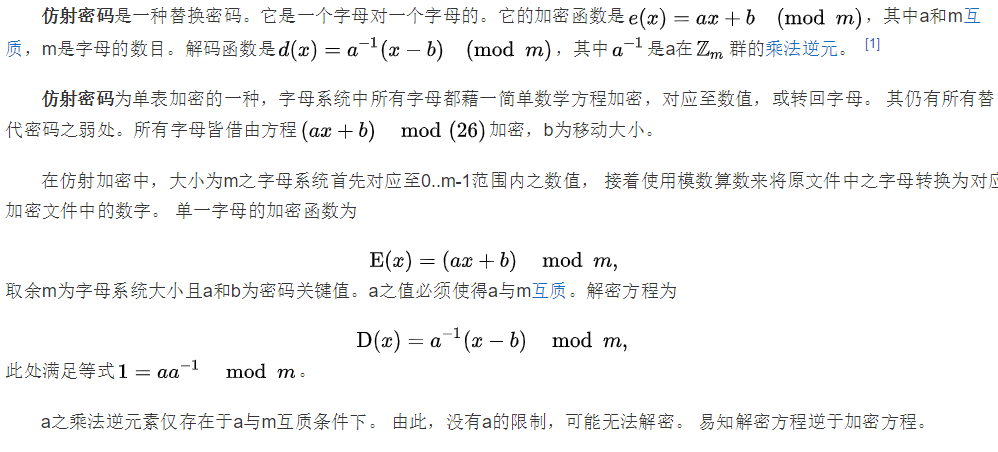只靠通识的数学知识，理解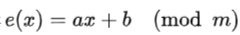是有难度

首先是计算优先级的疑惑，通过查资料确认是先计算前面的加法运算，然后再模，所以从非专业人士来说以下的通式形式会更好理解。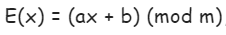这个式子对于代码来说应该是不难了，但是我要解的题目是类似这样的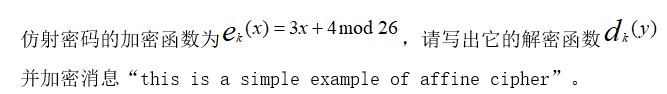那么怎么将字母作为可计算的值代入式子呢。参考这个文章

https://www.cnblogs.com/Mayfly-nymph/p/12394329 得知，以26个字母为编码系统为例，那么x代表的是字母在编码系统中的位序，

以 (3x+4)/mod 26为例，对 this字符进行加密的计算过程是这样

 明文 t h i s x 19 7 8 18 3x+4 61 25 28 58 Mod 26 9 25 2 6 密文 j z c g

了解这个之后，代码实现就很简单了，就是一个转换关系，如下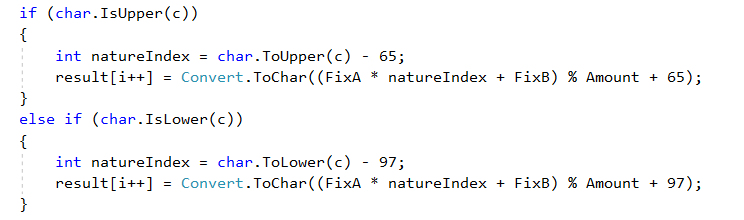解密函数的形式是这样的：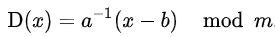𝑎−1表示是 a 关于m的乘法逆元，关于这个概念可以参考这里https://blog.csdn.net/mochounv/article/details/109254137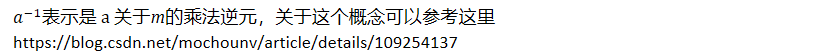简单来说就是𝑎−1amod26=1,就是一个最小与本身相乘再对26求模结果为1的数，因为a=3，所以这里很容易推算出 𝑎−1=9代码求是这样的：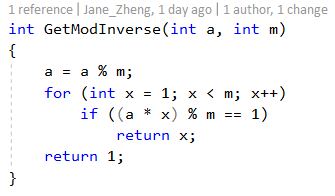所以得到这个系数之后 ，解密函数转成代码也就很简单了，如下：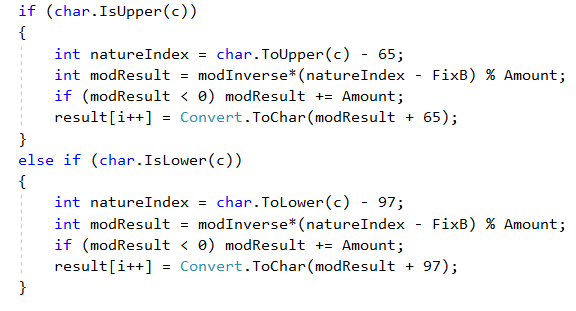所以文中提到的题目，根据以上代码转换函数计算结果如下：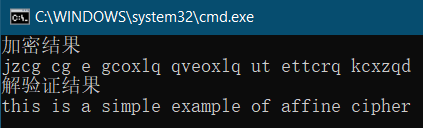源码可以从这里下载：

展开全文1024程序员节 仿射加密 仿射解密
• 本文实例为大家分享了java实现仿射密码加密解密的具体代码，供大家参考，具体内容如下加密：将明文转化为对应的数字，如 ‘a'-> 0, ‘b'->1,…,'1'->26,'2'->27,…然后将数字进行仿射运算，求取出来的...

本文实例为大家分享了java实现仿射密码加密解密的具体代码，供大家参考，具体内容如下

加密：将明文转化为对应的数字，如 ‘a'-> 0, ‘b'->1,…,'1'->26,'2'->27,…然后将数字进行仿射运算，求取出来的数字再转化为字符。即 密文=(K1*明文+K2)mod36

解密：密文转化为对应数字，然后进行仿射的逆运算，得到对应数字，然后将其转化为字符明文。解密 K3是K1的乘法逆元

import java.util.Scanner; public class Affine{ public static void main(String[] args) { char[] form = {'A', 'B', 'C', 'D', 'E', 'F', 'G', 'H', 'I', 'J', 'K', 'L', 'M', 'N', 'O', 'P', 'Q', 'R', 'S', 'T', 'U', 'V', 'W', 'X', 'Y', 'Z', '0' , '1', '2', '3', '4', '5', '6', '7', '8', '9'}; Scanner sc = new Scanner(System.in); System.out.println("请输入待加密的明文："); String MingWen = sc.nextLine(); MingWen=MingWen.toUpperCase();//将输入的明文全部大写 final int K1 = 103; final int K2 = 103; final int K3 = 31; int [] cipherNum=new int[MingWen.length()];//用来存储数字化的密文 encryption(MingWen,form,K1,K2,cipherNum); decryption(form,MingWen,K2,K3,cipherNum); } public static void encryption(String MingWen,char[] form,int K1,int K2,int[] cipherNum){ //第一步：将明文存入数组 char[] pla=new char[MingWen.length()]; for (int i = 0; i

以上就是本文的全部内容，希望对大家的学习有所帮助，也希望大家多多支持华域联盟。

展开全文java实现仿射密码
• C语言实现仿射密码实验 废话不多说直接上代码 #include<stdio.h> int main(){ int p,k1,k2;//仿射密钥 c=k1*m+k2 mod p 古典密码p=26 int N = 1000; char message[N+1]; printf("Input the message ...

C语言实现仿射密码实验
废话不多说直接上代码

#include<stdio.h>
int main(){
int p,k1,k2;//仿射密钥 c=k1*m+k2 mod p 古典密码p=26
int N = 1000;

char message[N+1];

printf("Input the message to process:");

//以回车（换行）作为字符串读取的结束,默认遇空格、回车、跳格键结束。

scanf("%[^\n]",message);

printf("\nThe message/plaintext is:%s",message);

int length=strlen(message);

char cipher[length],decipher[length];

int type;//加解密类型

printf("\n请选择加解密方式: 0--加密，1--解密：");

scanf("%d",&type);

if(type==0)
{
printf("对输入的文本进行加密处理：\n");
printf("\nPlease input the three affine cipher parameters：k1,k2,p,以逗号分隔:");

//读取数值时，默认以空格分隔，如需逗号则在%d后面指定格式：scanf("%d,%d",&k1,&k2);

scanf("%d,%d,%d",&k1,&k2,&p);

printf("\nThe input keys are: k1=%d , k2= %d, p= %d \n",k1,k2,p);

//getchar()在scanf()后，可以吸收回车符，以便输入下一个字符/串。特别是在后续需输入含空格的字符串时

getchar();
int i ;
for(i=0; i<length;i++)

{

if(message[i]>='a'&&message[i]<='z')

cipher[i]=(k1*(message[i]-'a')+k2)%p+'a';

else

if(message[i]>='A'&&message[i]<='Z')

cipher[i]=(k1*(message[i]-'A')+k2)%p+'A';

else{

cipher[i]=message[i];
}
}
cipher[length]='\n';//字符串时及时添加结束标志以防额外增加数组长度。
printf("消息加密前后对比如下：\n");

printf("The Input  Text is: %s.\n",message);

printf("The Output Text is: %s.\n",cipher);

file_write(cipher);//打印生成一个加密后的文本文件作为存储以方便后续的解密过程
}
else{
printf("文本将进行解密处理：\n");
int s,t;

int reverse;//k1的逆元

int i;
printf("\nPlease input the three affine cipher parameters：k1,k2,p,以逗号分隔:");

//读取数值时，默认以空格分隔，如需逗号则在%d后面指定格式：scanf("%d,%d",&k1,&k2);

scanf("%d,%d,%d",&k1,&k2,&p);

printf("\nThe input keys are: k1=%d , k2= %d, p= %d \n",k1,k2,p);
reverse=ojld(k1,p,s,t);

for(i=0; i<length;i++){

if(message[i]>='a'&&message[i]<='z')
{
decipher[i]=(reverse*((cipher[i]-'a')-k2) )%p+'a';
}
else
if(message[i]>='A'&&message[i]<='Z')
{
decipher[i]=(reverse*((cipher[i]-'A')-k2))%p+'A';
}
else
decipher[i]=message[i];

}
printf("经过解密内容为：%s.\n",decipher);
}

}

void file_write(char*cipher)
{

int i;

FILE *outfile;

outfile = fopen("加密.txt","w");

if(outfile==NULL)

{

printf("Can't open the file!\n");

}

fprintf(outfile,"%s",cipher);

fclose(outfile);

}

int gcd(int a,int b){//欧几里得算法

if(a<b){

int t=b;

b=a;

a=t;
}

while(a%b!=0){

int r=a%b;

a=b;

b=r;

r=a%b;
}

return b;

}
int ojld(int r0,int r1,int s,int t){//欧几里得扩展算法

int R0=r0,R1=r1;

if(r0==0||r1==0){

printf("ERROR:One of the two input numbers is zero.\n");

return 0;

}

int q=0,r2=0,s0=1,s1=0,s2=0,t0=0,t1=1,t2=0;

while(r1!=0){

q=r0/r1;

r2=r0%r1;

s2=s0-s1*q;//计算新s

t2=t0-t1*q;//计算新t

//更新r0,r1,s0,s1,t0,t1
r0=r1;r1=r2;

s0=s1;s1=s2;

t0=t1;t1=t2;//保存s2,t2的值，以备下次使用
}
if(r0==1){

s0=(R1+(s0%R1))%R1;

t0=(R0+(t0%R0))%R0;
//printf("Afterwards [P+(x mod P)]mod P:s0=%d,t0=%d.\n",s0,t0);
printf("k1的逆元：%d.\n",s0);

s=s0;

t=t0;
//printf("THE GCD is:%d UNEQUAL TO 1，NO INVERSE EXITS.\n",r0);
return s0;

}

}

展开全文c语言 加密解密
• 主要介绍了基于Python解密仿射密码,文中通过示例代码介绍的非常详细，对大家的学习或者工作具有一定的参考学习价值,需要的朋友可以参考下
• 主要介绍了Python实现仿射密码的思路详解,本文通过实例代码给大家介绍的非常详细，对大家的学习或工作具有一定的参考借鉴价值，需要的朋友可以参考下
• 加密程序 解密程序 待加密文本 待解密文本 加密函数: Y=（AX+B）%26 解密函数: X=（A的逆元）*（Y-B）%26 加密程序 #include&lt;stdio.h&gt; int main(){ FILE *fp = ("fangshe1.txt"...仿射密码
• 仿射密码 使用Vue.js进行仿射密码加密！ #NotFancyAppChallenge 检查以下应用程序： 如果您要检查此应用程序，请单击JavaScript
• 加法密码和乘法密码结合就构成仿射密码仿射密码加密算法是： C= Ek(m)=(k1*m+k2) mod n 在这里我们规定n=26;其中明文信息为26个小写字母（az）集合，密文为26个大写字母(AZ)集合，然后在对大写字母作进一步操作...算法 加密解密
• Java实现仿射密码加密解密

千次阅读 热门讨论 2019-01-17 13:49:36
Java实现仿射密码加密解密 仿射密码 加密：将明文转化为对应的数字，如 ‘a’-&gt; 0, ‘b’-&gt;1,…,’1’-&gt;26,’2’-&gt;27,…然后将数字进行仿射运算，求取出来的数字再转化为字符。即 密文=...
• 基于C语言，实现仿射密码算法，随机生成密钥a、b，加密和解密程序对任意满足条件的a、b都能够进行处理。
• 仿射密码为单表加密的一种，字母系统中所有字母都藉一简单数学方程加密，对应至数值，或转回字母。 其仍有所有替代密码之弱处。所有字母皆借由方程 加密，b为移动大小。 在仿射加密中，大小为m之字母系统首先对应至0...
• 仿射密码加密

2018-11-10 11:56:29
加密算法 int encode(FILE *m,FILE *c,int a,int b){ char ch = fgetc(m); char r; while(ch != EOF){ if(ch &gt;='A' &amp;&amp; ch &lt;= 'Z'){ //大写字母加密 r=((ch-'A')*a+b...古典密码
• 实验3：仿射密码加密 【实验步骤及描述】 题目要求： 利用放射密码（双换位密码）加密消息 We are all together 行变换为2413，列变换为3124 1.使用VScode自己编写C++程序完成实验 双换位密码使用二维数组存放数据，...信息安全 物联网
• http://blog.csdn.net/amazinguu/article/details/51416681Android 加密
• 用matlab来实现仿射密码，主要是现代密码学课上的实验。仿射密码
• 仿射密码为单表加密的一种，字母系统中所有字母都藉一简单数学方程加密，对应至数值，或转回字母mima
• gcd(k1,n)=1为仿射密码具有可逆性的条件 35 // TODO Auto-generated method stub 36 int k=0; // 37 do { 38 k=k1% n; 39 k1= n; 40 n= k; 41 } while (k!=0 ); 42 return k1; 43 } ...
• 仿射密码的加密解密

千次阅读 2018-12-19 22:45:24
仿射密码的加解密代码 因为加密的为英文字母以及数字,所以模数选择26+10=36. 再选一个k1为一个与36互素的数字. 再任意选一个k2 求除k1在模36下的逆元k3.(欧几里得除法,代码也可实现,这里没写,直接给出) 加密为: c=...仿射密码 密码学实验
• 仿射密码的加密与解密

千次阅读 2020-11-27 17:01:52
仿射密码为单表加密的一种，字母系统中所有字母都藉一简单数学方程加密，对应至数值，或转回字母。它是一种替换密码，利用加密函数一个字母对一个字母的加密. 加密函数 加密函数是 E(x)= (ax + b) (mod m)，其中，a...加密解密 信息安全 python
• 仿射密码加密算法

千次阅读 2013-09-21 10:21:46
加法密码和乘法密码结合就构成仿射密码仿射密码加密和解密算法是：  C= Ek(m)=(k1m+k2) mod n  M= Dk(c)=k3(c- k2) mod n（其中（k3 ×k1）mod26 = 1）    仿射密码具有可逆性的条件是gcd(k1, n)=1。......